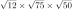Question Pool New Numbers

# New Numbers - SAMAGRA Question Pool & Answers | Class 9 English Medium

Kerala Syllabus SAMAGRA SCERT SAMAGRA Question Pool for Class 9 English Medium Maths New NumbersQn 1.ABCD is a rectangle AB =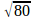cm.,AD =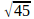cm., a) What is the perimeter of ABCD ? b) Find the area of ABCD

Get Free Study Materials + 1 Week Free Trial of BrainsPrep Class 9 English Medium Tuition

Qn 2.

In the figure AC = 5 cm. , <B = 90°, <C = 45°

What is the length of AB ?.( Correct to two decimal places)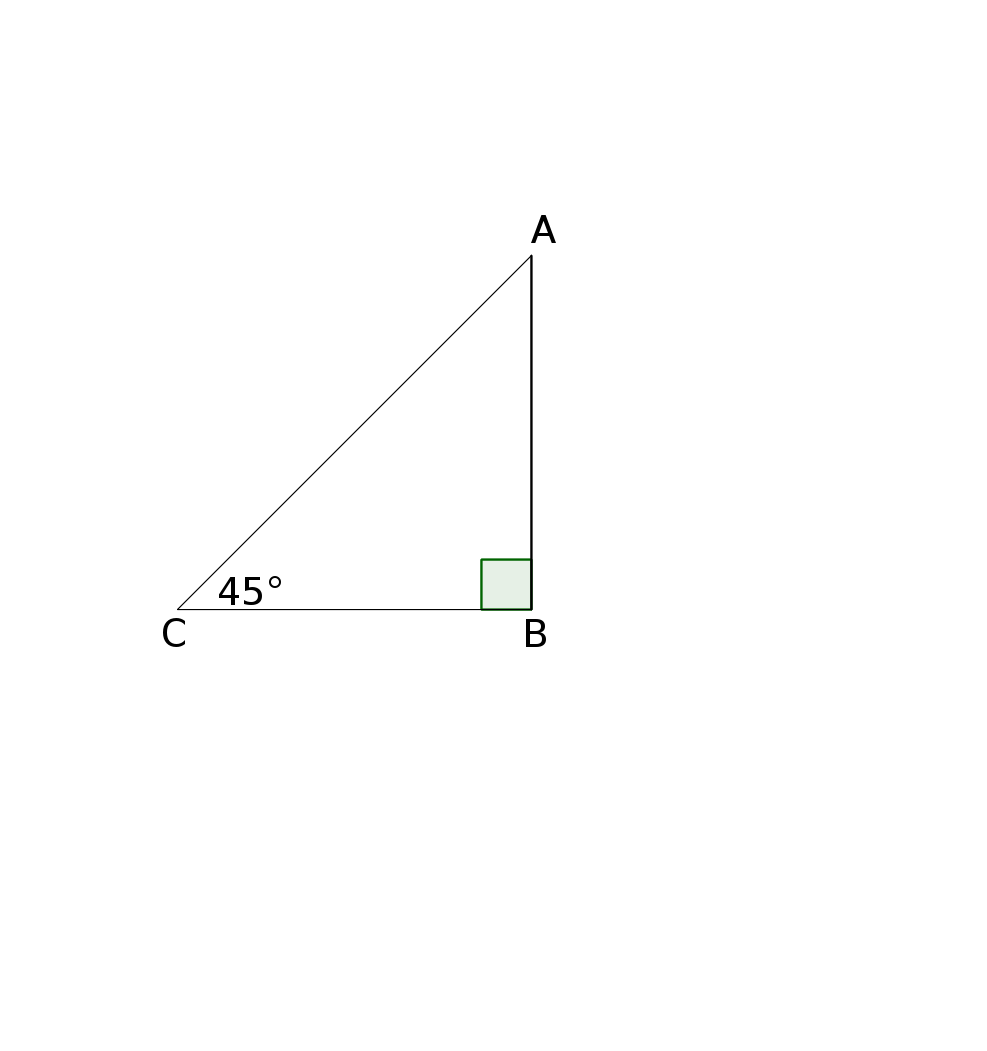Get Free Study Materials + 1 Week Free Trial of BrainsPrep Class 9 English Medium Tuition

Qn 3.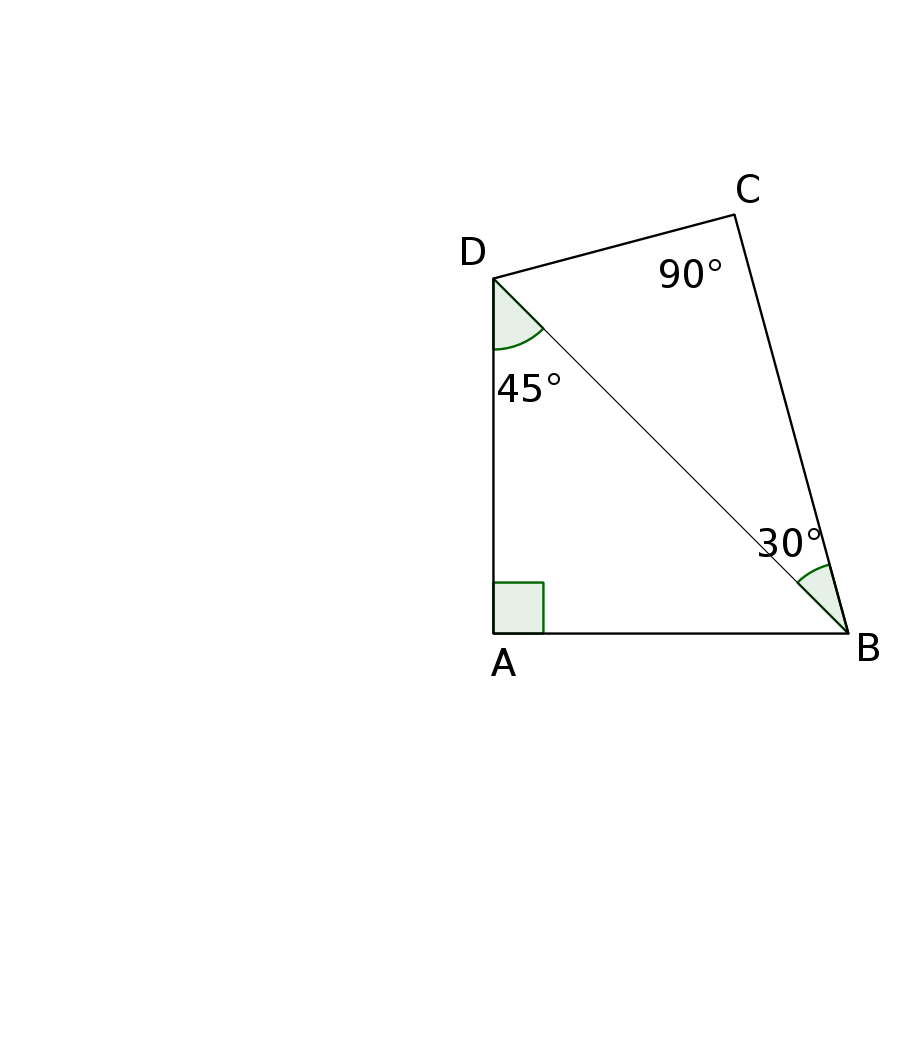In the figure < A = <C = 90° , <ADB = 45° , <DBC = 30° ,BD = 8സെ.മീ. ആണ്

a) What is the length of AB ?

b) What is the perimeter  of ABCD?

c) What is the area of ABCD ?

Get Free Study Materials + 1 Week Free Trial of BrainsPrep Class 9 English Medium Tuition

Qn 4.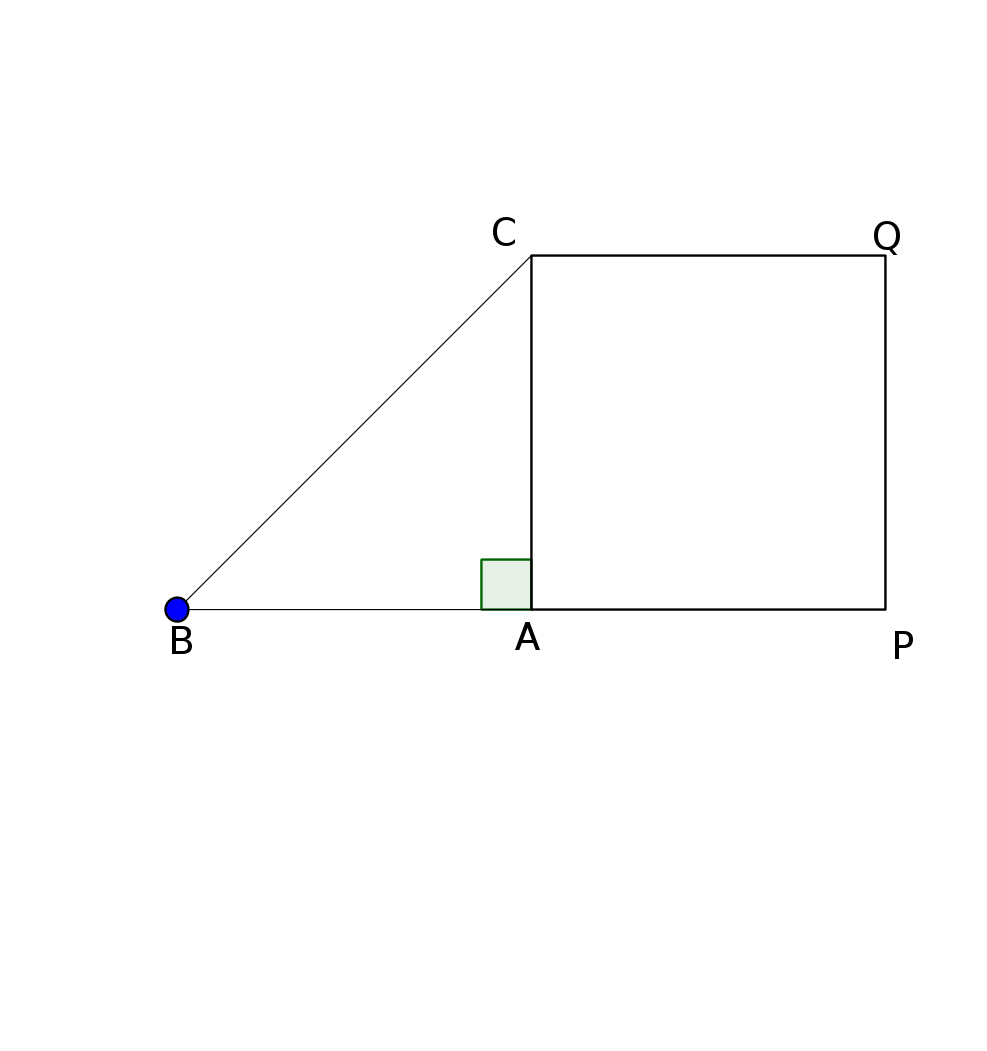In the figure AB = 2 cm. BC = 3 cm., <A= 90°

a) What is the length of AC ?

b) What is area of the square APQC

c) Draw a square of area 5 sq. cm.

Get Free Study Materials + 1 Week Free Trial of BrainsPrep Class 9 English Medium Tuition

Qn 5.

ABCD is a square. length of one diagonal is 5 cm.

a) What is the length of AB ?.

b) Find the perimeter of ABCD

c) Find the area of ABCDGet Free Study Materials + 1 Week Free Trial of BrainsPrep Class 9 English Medium Tuition

Qn 6.

In the figure ABCD is a square and and P,Q,R,S are the mid points of the sides

a)If AB = 2 cm. , what is the area ofABCD?

b) What is the area of PQRS?c) What is the length of PQ?

Get Free Study Materials + 1 Week Free Trial of BrainsPrep Class 9 English Medium Tuition

Qn 7.

a) (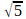+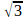) (-) = ..............

b)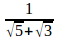+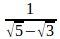= .................

c)x= ..............

Get Free Study Materials + 1 Week Free Trial of BrainsPrep Class 9 English Medium Tuition

Qn 8.

a) What is the product of a number and its reciprocal ?

b) Find (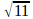+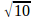) (-)

c) Write the reciprocal of+Get Free Study Materials + 1 Week Free Trial of BrainsPrep Class 9 English Medium Tuition

Qn 9.

Breadth of a rectangle is 4 cm.smaller than that of its legth. If the length is+ 2 cm.

b) Find the perimeter and area of the rectangle

Get Free Study Materials + 1 Week Free Trial of BrainsPrep Class 9 English Medium Tuition

Qn 10.

Which among the following is not a natural number?

a) (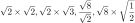)

b)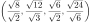Get Free Study Materials + 1 Week Free Trial of BrainsPrep Class 9 English Medium Tuition

Qn 11.

In figure, a side of the smallest square is 1 centimetre. Another square is drawn on its diagonal, next square on its diagonal,...Among the squares thus obtained

a) Find the area of second square.

b) Write the areas of the first 6 squares.

c) Write the sides of the first 6 squares.

c) Is the side of 10th square a fraction? What about the side of 11th square?

d) Write the perimeter of the first 4 squares.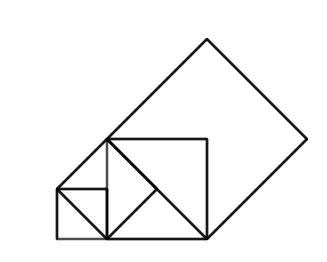Get Free Study Materials + 1 Week Free Trial of BrainsPrep Class 9 English Medium Tuition

Qn 12.

We can write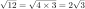and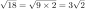a)Write the follo wing numbers like this

(i)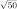(ii)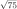(iii)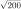b)Simplify

(i)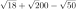(ii)